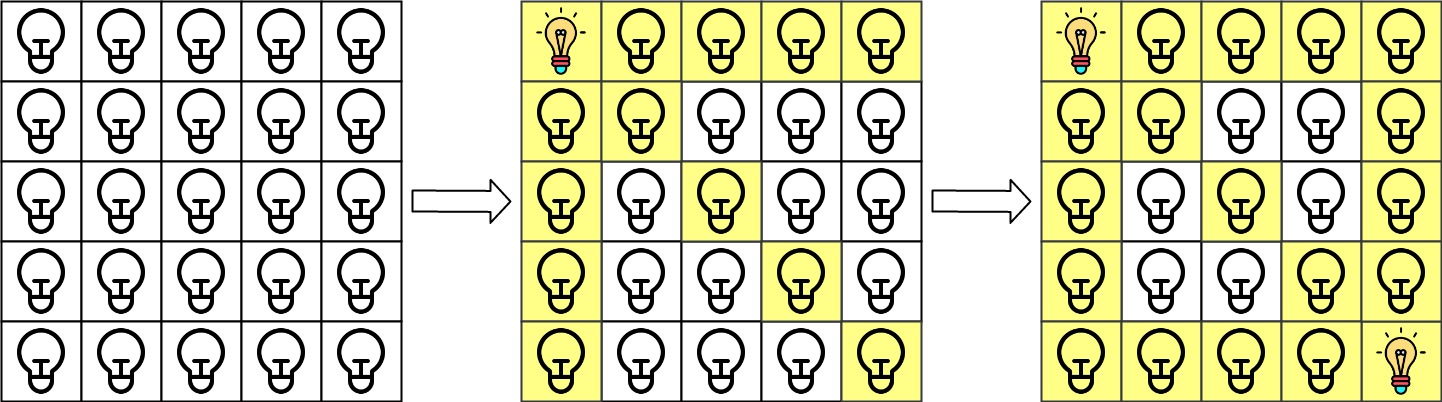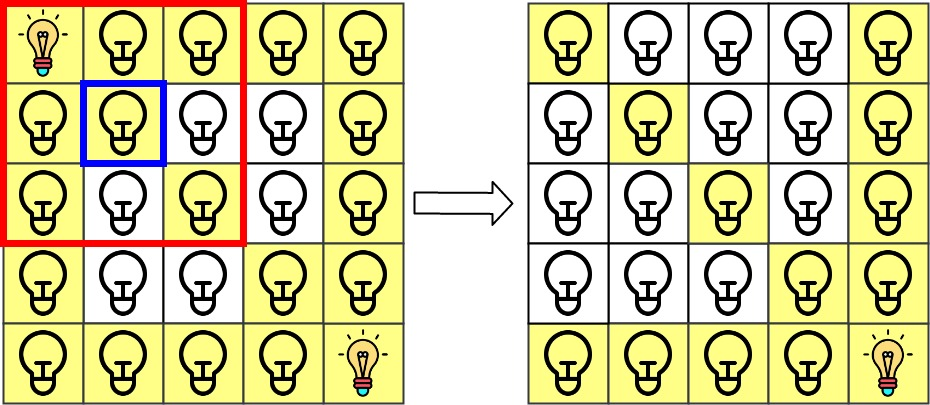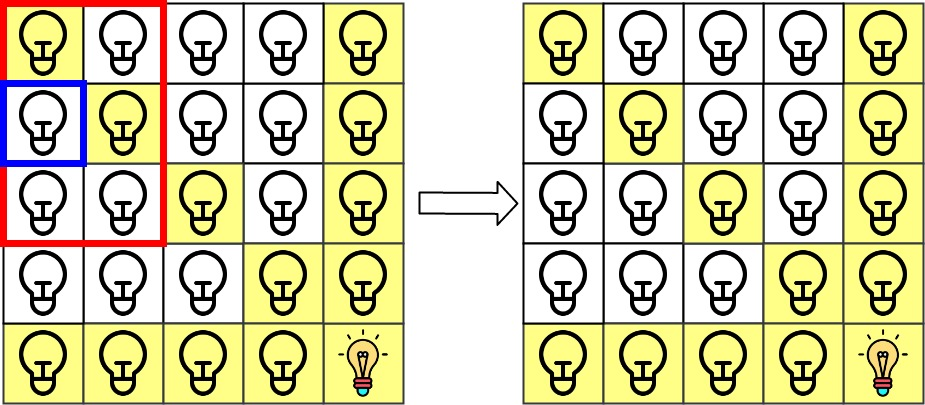## Algorithm

Problem Name: 1001. Grid Illumination

There is a 2D `grid` of size `n x n` where each cell of this grid has a lamp that is initially turned off.

You are given a 2D array of lamp positions `lamps`, where `lamps[i] = [rowi, coli]` indicates that the lamp at `grid[rowi][coli]` is turned on. Even if the same lamp is listed more than once, it is turned on.

When a lamp is turned on, it illuminates its cell and all other cells in the same row, column, or diagonal.

You are also given another 2D array `queries`, where `queries[j] = [rowj, colj]`. For the `jth` query, determine whether `grid[rowj][colj]` is illuminated or not. After answering the `jth` query, turn off the lamp at `grid[rowj][colj]` and its 8 adjacent lamps if they exist. A lamp is adjacent if its cell shares either a side or corner with `grid[rowj][colj]`.

Return an array of integers `ans`, where `ans[j]` should be `1` if the cell in the `jth` query was illuminated, or `0` if the lamp was not.

Example 1:```Input: n = 5, lamps = [[0,0],[4,4]], queries = [[1,1],[1,0]]
Output: [1,0]
Explanation: We have the initial grid with all lamps turned off. In the above picture we see the grid after turning on the lamp at grid then turning on the lamp at grid.
The 0th query asks if the lamp at grid is illuminated or not (the blue square). It is illuminated, so set ans = 1. Then, we turn off all lamps in the red square.The 1st query asks if the lamp at grid is illuminated or not (the blue square). It is not illuminated, so set ans = 0. Then, we turn off all lamps in the red rectangle.```

Example 2:

```Input: n = 5, lamps = [[0,0],[4,4]], queries = [[1,1],[1,1]]
Output: [1,1]
```

Example 3:

```Input: n = 5, lamps = [[0,0],[0,4]], queries = [[0,4],[0,1],[1,4]]
Output: [1,1,0]
```

Constraints:

• `1 <= n <= 109`
• `0 <= lamps.length <= 20000`
• `0 <= queries.length <= 20000`
• `lamps[i].length == 2`
• `0 <= rowi, coli < n`
• `queries[j].length == 2`
• `0 <= rowj, colj < n`

## Code Examples

### #1 Code Example with Javascript Programming

```Code - Javascript Programming```

``````
const gridIllumination = function (N, lamps, queries) {
const rowMap = new Map()
const colMap = new Map()
const hillMap = new Map()
const daleMap = new Map()
const litMap = new Map()
const direction = [
[0, 0],
[0, 1],
[1, 0],
[-1, 0],
[0, -1],
[-1, -1],
[1, 1],
]
//map what areas are lit
for (let [x, y] of lamps) {
insert(rowMap, x)
insert(colMap, y)
insert(hillMap, x + y)
insert(daleMap, x - y)
litMap.set(N * x + y, true)
}
const result = new Array(queries.length).fill(0)
let count = 0
for (let [x, y] of queries) {
if (
rowMap.get(x) > 0 ||
colMap.get(y) > 0 ||
hillMap.get(x + y) > 0 ||
daleMap.get(x - y) > 0
) {
result[count] = 1
}
for (let [i, j] of direction) {
let newX = x + i
let newY = y + j
if (litMap.has(N * newX + newY)) {
decrease(rowMap, newX)
decrease(colMap, newY)
decrease(hillMap, newX + newY)
decrease(daleMap, N * newX + newY)
litMap.delete(N * newX + newY)
}
}
count++
}
return result
}
const insert = (map, value) => {
if (map.has(value)) {
map.set(value, map.get(value) + 1)
} else {
map.set(value, 1)
}
}
const decrease = (map, value) => {
if (map.has(value)) {
map.set(value, map.get(value) - 1)
}
}
``````
Copy The Code &

Input

cmd
n = 5, lamps = [[0,0],[4,4]], queries = [[1,1],[1,0]]

Output

cmd
[1,0]

### #2 Code Example with Python Programming

```Code - Python Programming```

``````
class Solution:
def gridIllumination(self, N: int, lamps: List[List[int]], queries: List[List[int]]) -> List[int]:
lampsOn = set()
rowsOn = collections.defaultdict(int)
colsOn = collections.defaultdict(int)
diagOnTopLeftBottomRight = collections.defaultdict(int)
diagOnBottomLeftTopRight = collections.defaultdict(int)
for r, c in lamps:
rowsOn[r] += 1
colsOn[c] += 1
diagOnTopLeftBottomRight[c-r] += 1
diagOnBottomLeftTopRight[c+r-N] += 1

result = []
for r, c in queries:
if rowsOn[r] > 0 or colsOn[c] > 0 or diagOnTopLeftBottomRight[c-r] > 0 or diagOnBottomLeftTopRight[c+r-N] > 0:
result.append(1)
else:
result.append(0)
adjacentLamps = [(r, c), (r, c-1), (r, c+1), (r-1, c), (r-1, c-1), (r-1, c+1), (r+1, c), (r+1, c-1), (r+1, c+1)]
if (r, c) in lampsOn:
rowsOn[r] -= 1
colsOn[c] -= 1
diagOnTopLeftBottomRight[c-r] -= 1
diagOnBottomLeftTopRight[c+r-N] -= 1
return result
``````
Copy The Code &

Input

cmd
n = 5, lamps = [[0,0],[4,4]], queries = [[1,1],[1,0]]

Output

cmd
[1,0]